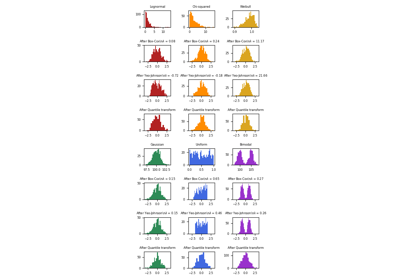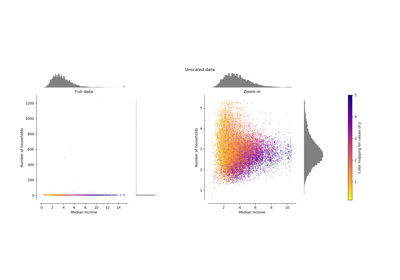# sklearn.preprocessing.PowerTransformer¶

class sklearn.preprocessing.PowerTransformer(method=’yeo-johnson’, standardize=True, copy=True)[source]

Apply a power transform featurewise to make data more Gaussian-like.

Power transforms are a family of parametric, monotonic transformations that are applied to make data more Gaussian-like. This is useful for modeling issues related to heteroscedasticity (non-constant variance), or other situations where normality is desired.

Currently, PowerTransformer supports the Box-Cox transform and the Yeo-Johnson transform. The optimal parameter for stabilizing variance and minimizing skewness is estimated through maximum likelihood.

Box-Cox requires input data to be strictly positive, while Yeo-Johnson supports both positive or negative data.

By default, zero-mean, unit-variance normalization is applied to the transformed data.

Read more in the User Guide.

Parameters: method : str, (default=’yeo-johnson’) The power transform method. Available methods are: ‘yeo-johnson’ [Rf3e1504535de-1], works with positive and negative values ‘box-cox’ [Rf3e1504535de-2], only works with strictly positive values standardize : boolean, default=True Set to True to apply zero-mean, unit-variance normalization to the transformed output. copy : boolean, optional, default=True Set to False to perform inplace computation during transformation. lambdas_ : array of float, shape (n_features,) The parameters of the power transformation for the selected features.

power_transform
Equivalent function without the estimator API.
QuantileTransformer
Maps data to a standard normal distribution with the parameter output_distribution='normal'.

Notes

NaNs are treated as missing values: disregarded in fit, and maintained in transform.

For a comparison of the different scalers, transformers, and normalizers, see examples/preprocessing/plot_all_scaling.py.

References

 [Rf3e1504535de-1] I.K. Yeo and R.A. Johnson, “A new family of power transformations to improve normality or symmetry.” Biometrika, 87(4), pp.954-959, (2000).
 [Rf3e1504535de-2] G.E.P. Box and D.R. Cox, “An Analysis of Transformations”, Journal of the Royal Statistical Society B, 26, 211-252 (1964).

Examples

>>> import numpy as np
>>> from sklearn.preprocessing import PowerTransformer
>>> pt = PowerTransformer()
>>> data = [[1, 2], [3, 2], [4, 5]]
>>> print(pt.fit(data))
PowerTransformer(copy=True, method='yeo-johnson', standardize=True)
>>> print(pt.lambdas_)
[ 1.386... -3.100...]
>>> print(pt.transform(data))
[[-1.316... -0.707...]
[ 0.209... -0.707...]
[ 1.106...  1.414...]]


Methods

 fit(self, X[, y]) Estimate the optimal parameter lambda for each feature. fit_transform(self, X[, y]) get_params(self[, deep]) Get parameters for this estimator. inverse_transform(self, X) Apply the inverse power transformation using the fitted lambdas. set_params(self, \*\*params) Set the parameters of this estimator. transform(self, X) Apply the power transform to each feature using the fitted lambdas.
__init__(self, method=’yeo-johnson’, standardize=True, copy=True)[source]
fit(self, X, y=None)[source]

Estimate the optimal parameter lambda for each feature.

The optimal lambda parameter for minimizing skewness is estimated on each feature independently using maximum likelihood.

Parameters: X : array-like, shape (n_samples, n_features) The data used to estimate the optimal transformation parameters. y : Ignored self : object
get_params(self, deep=True)[source]

Get parameters for this estimator.

Parameters: deep : boolean, optional If True, will return the parameters for this estimator and contained subobjects that are estimators. params : mapping of string to any Parameter names mapped to their values.
inverse_transform(self, X)[source]

Apply the inverse power transformation using the fitted lambdas.

The inverse of the Box-Cox transformation is given by:

if lambda == 0:
X = exp(X_trans)
else:
X = (X_trans * lambda + 1) ** (1 / lambda)


The inverse of the Yeo-Johnson transformation is given by:

if X >= 0 and lambda == 0:
X = exp(X_trans) - 1
elif X >= 0 and lambda != 0:
X = (X_trans * lambda + 1) ** (1 / lambda) - 1
elif X < 0 and lambda != 2:
X = 1 - (-(2 - lambda) * X_trans + 1) ** (1 / (2 - lambda))
elif X < 0 and lambda == 2:
X = 1 - exp(-X_trans)

Parameters: X : array-like, shape (n_samples, n_features) The transformed data. X : array-like, shape (n_samples, n_features) The original data
set_params(self, **params)[source]

Set the parameters of this estimator.

The method works on simple estimators as well as on nested objects (such as pipelines). The latter have parameters of the form <component>__<parameter> so that it’s possible to update each component of a nested object.

Returns: self
transform(self, X)[source]

Apply the power transform to each feature using the fitted lambdas.

Parameters: X : array-like, shape (n_samples, n_features) The data to be transformed using a power transformation. X_trans : array-like, shape (n_samples, n_features) The transformed data.

## Examples using sklearn.preprocessing.PowerTransformer¶Map data to a normal distributionCompare the effect of different scalers on data with outliers